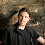## Thursday, November 19, 2009

### «Double 0 Degrees»

The temperature of a hypothetical object today is 0 degrees Celsius. Tomorrow the object will be twice that. What temperature will it be tomorrow? I'll give you a hint: it's not 64 degrees Fahrenheit.

Seeing as the Celsius scale is based on the arbitrary freezing point of water, and not the actual total amount of heat energy, we need to convert Celsius to to a scale that is based off the absolute heat energy: Kelvin. In the Kelvin scale, there is no such thing as below 0. 0 Kelvin is called absolute zero, where there is literally no heat energy.

0 degrees Celsius is 273.15 in the Kelvin scale. We can then double 273.15 and get 546.3 Kelvin. It can be converted back to Celsius as 273.15 degrees.

This isn't just a metric thing, either. It works out in the Rankine scale, which is also based on absolute heat energy. 32 degrees Fahrenheit (equal to 0°C) is 491.67 Rankine, double that and you get 983.34 Rankine. Convert it back to Fahrenheit and you get 523.67 degrees Fahrenheit. 523.67 degrees Fahrenheit is exactly equal to 273.15 degrees Celsius.

Makes you appreciate how much heat energy is really in the objects around us.

1.Whoa! I never realized freezing temperature was so hot!
Vid

2.That is entirely too much for my brain at 8 50am

3.Heres a trick question. if celsius is 0 degrees freezing point and if you double it you should get 0 but the converting makes it 273.15 heat energy at double but it would still be 0.

4.@ Stefan: That's if you double the number 0. However, temperature is a way of measuring heat energy. So you'd want to double the heat energy, not just the number 0.

Thanks for taking the time to comment.